Courses

# Regulation & Efficiency Notes | EduRev

## Electrical Engineering (EE) : Regulation & Efficiency Notes | EduRev

The document Regulation & Efficiency Notes | EduRev is a part of the Electrical Engineering (EE) Course Electrical Machines for Electrical Engg..
All you need of Electrical Engineering (EE) at this link: Electrical Engineering (EE)

What is voltage Regulation?
The term voltage regulation identifies the characteristic of the voltage change in the transformer with loading.
The voltage regulation of the transformer is defined as the arithmetical difference in the secondary terminal voltage between no-load (I2 = 0) and full rated load (I2 = I2fl) at a given power factor with the same value of primary voltage for both rated load and no-load.
The numerical difference between no-load and full-load voltage is called inherent voltage regulation.
Inherent voltage regulation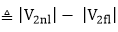Where V2fl = rated secondary terminal voltage at rated load.
V2nl = no load secondary terminal voltage with the same value of primary voltage for both rated load and no load.
Per unit voltage regulation at full load is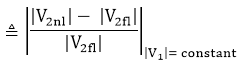Percent voltage regulation at full load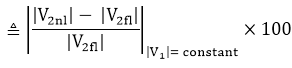We can say that voltage regulation is an important measure for the performance of the transformer. We can specify the limits of the transformer in terms of voltage regulation.

Voltage Regulation in terms of primary values:
Per unit voltage regulation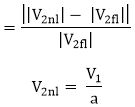∴pu voltage regulation is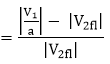Calculation of Voltage Regulation
We can calculate the voltage regulation of a transformer in terms of circuit parameters. The equivalent circuit diagram of a transformer referred to secondary is shown below.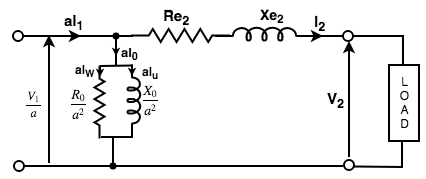Figure: Equivalent circuit of the transformer referred to the secondary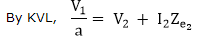To calculate the voltage regulation we have to follow the steps given below:
1. We have to take V2 as the reference Phasor
∴ V2 = V2∠0°= V2 + j0

2. Write I2 in Phasor form
For lagging power-factor CosФ2
I2 = I2 ∠- Ф2 = I2Cos Ф2? jI2 sinФ2
I2 = I2 ∠+ Ф2 = I2Cos Ф2 + jI2 sinФ2
For unity power factor
I2 = I2∠0° = I2 +j0

3. Calculate Ze2
Ze2 = Re2 + jXe2

4. Calculate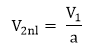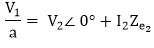5. Now we will calculate the voltage regulation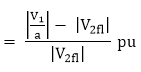Or we can say that it is the ratio of the difference between the voltages at no load and full load to the voltage at full load.

What is Transformer Efficiency?
Transformer efficiency (η) can be explained as the ratio of the output power to the input power.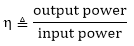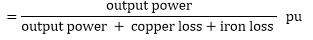Therefore the per unit efficiency at load current 12 and power factor cos Ф2 will be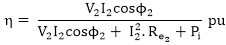And the per unit efficiency at full load is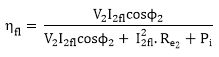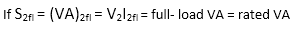Then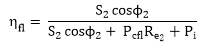As we know that in a transformer there is no rotational part so there are no rotational losses such as windings and frictional losses in a rotating machine. Therefore, we can obtain a maximum efficiency as high as 99% in a well-designed transformer.

What is all day (or energy) efficiency of a transformer?
The ratio of the energy output of 24-hour to the energy input of a 24-hour period is called the All-day efficiency of a transformer.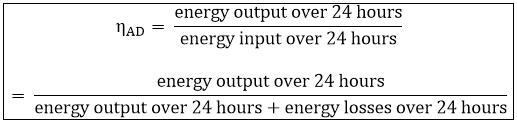If we know the load cycle of the transformer, then all day efficiency can easily be determined.

Offer running on EduRev: Apply code STAYHOME200 to get INR 200 off on our premium plan EduRev Infinity!

## Electrical Machines for Electrical Engg.

22 videos|41 docs|26 tests

,

,

,

,

,

,

,

,

,

,

,

,

,

,

,

,

,

,

,

,

,

;